# PWM Input Control for S12Z

cancel
Showing results for
Did you mean:

# Introduction

In many old-fashioned automotive motor control systems, PWM command communication is still preferred over LIN or CAN. Even for in-lab application debugging, it is very convenient to use PWM command communication to control the motor placed in a test-bench or in an acoustic or thermal chamber, especially when the BDM debugger or the SCI/RS232 communication is not available.

PWM control is usually specified on the OEM level and it is not provided as a public standard. Nevertheless, adjustable software driver can be created to help the PWM command signal to be detected. The following code has been developed for S12ZVM, but it can be easily adopted to fit in whatever device with a timer input capture feature.

Hardware specification may be different for many cases and the implementation is the matter of proper design case-by-case and may be a very easy task for a skilled designer. However, software design can be abstracted and reused for different hardware implementation. In order to help you use the PWM control signal, the following description is provided.

The example provided is targeting NXP MagniV S12ZVML devices, but may be used along with any device accordingly. The S12ZVML MCU contains LIN physical layer which will be used as PWM input in our case.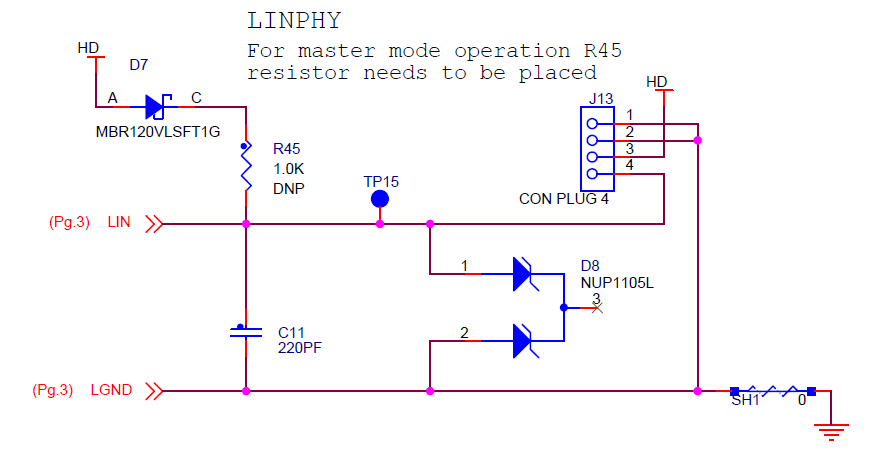# PWM Signal Specification

Since there is no public standard specification on PWM signal, let's target a general case with adjustable parameters. Since the PWM control signal is being replaced by LIN communication, the specification may be based on 5.5V to 18V voltage range with nominal 12V level. PWM frequency varies from 10 Hz to 1 kHz (or more), but it really depends on the requirements of the application, compatibility, etc. Duty-cycle range of the PWM signal might be from 15% to 90% from stand-by to full speed. Hysteresis at low duty-cycle range (between 10 and 15%) prevents the system from periodic on/off switching at the lowest duty-cycle. Duty-cycles outside of this range may be identified as not valid and appropriate action may be taken (e.g. full speed command to an engine cooling fan).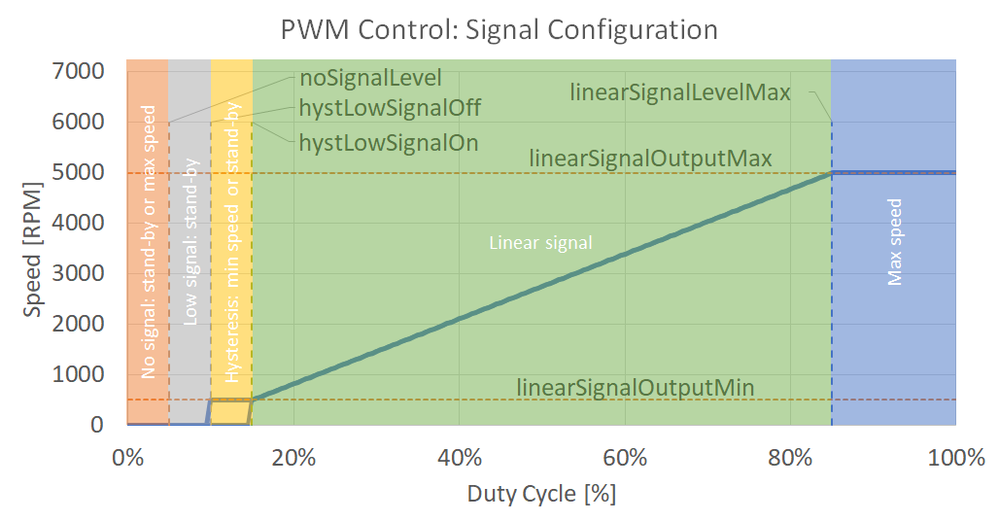The signal definition should consider some specific values to help with the signal reading. Following values are defined:

#### Description

noSignalLevelNo signal detected below this duty cycle
noSignalOutputOutput on "no signal" detected
lowSignalOutputOutput on "low signal" detected
hystLowSignalOffLow level of the hysteresis. Smaller duty-cycle means "low signal" state, higher duty-cycle enters the hysteresis range (min speed or stand-by)
hystLowSignalOnHigh level of the hysteresis. Smaller duty-cycle means "hysteresis area: min speed or stand-by", higher duty-cycle means "run"  in the linear signal range, with the speed given by the duty-cycle.
linearSignalOutputMinLow level of the linear mode
linearSignalLevelMaxHigh level of the linear mode

Considering normal operation in stand-by mode, the duty-cycle is between noSignalLevel and hystLowSignalOff. In order to add some distortion-proof feature, the duty-cycle can go up to the hystLowSignalOn and the stand-by mode would be still detected. In order to engage the "run" state, the duty-cycle has to exceed the hystLowSignalOn value and the linear area is reached. Corresponding output is calculated to meet the linearSignalOutputMin at the hystLowSignalOn value and linearSignalOutputMax at the linearSignalLevelMax. System keeps the output at linearSignalOutputMax for duty-cycles higher than linearSignalLevelMax. When slowing down, reaching the hystLowSignalOn sets the output to linearSignalOutputMin and keeps this output until the hystLowSignalOff is reached. This way, the hysteresis feature is provided in order to prevent motors from randomly switch on and off in case the PWM signal is noisy. Lowering the duty-cycle below the hystLowSignalOff, zero output is set, which represents the stand- by mode. Duty-cycles below the noSignalLevel are treated as not valid and noSignalOutput is engaged (which can be set to zero or to max, according to the application requirements).

## Requirements on Timer

In order to correctly detect PWM signal frequency and duty-cycle, hardware timer with input capture feature and rising/falling edges detection shall be used. Timing and chaining of multiple timer channels may be needed to increase the frequency range or duty-cycle resolution. Following example will be simplified to use just one 16-bit timer channel (timer module TIM16B4CV3 used in S12ZVML128). The input of the timer shall be routed to an input pin, on which the PWM signal will be sensed. Integrated LIN physical layer module of S12ZVML128 device can be used for signal conditioning since the signal voltage range is up to 18V. In the example, LINPhy module has to be enabled and the LPRXD0 signal is routed to the timer T0 input capture channel 3 by setting "T0IC3RR1-0" of the MODRR2 register to 0b01. Input capture and timer overflow interrupts are used to detect correct or faulty signal.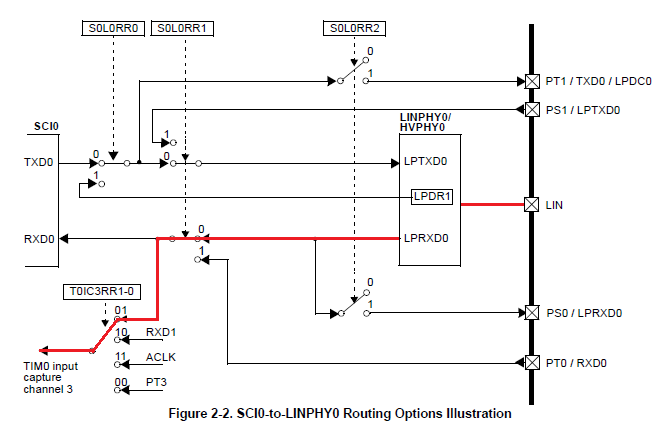## Requirements on Application Software

PWM signal detection is called within the Timer Input Capture interrupt. This way, the duty-cycle is calculated immediately. Signal lost event is detected within the Timer overflow interrupt. It is recommended that the detected duty-cycle is filtered by a low-pass filter (moving average or IIR), which is usually called within some real-time interrupt routine, such as PWM reload or ADC end-of-scan interrupt. Reading of the output value can be done anytime within the code, e.g. within a speed loop to read the demanded speed command.

# Algorithm Description

## Frequency and Duty-cycle Detection

Frequency of PWM signal is given by the time between two consecutive rising edges or two falling edges. The duty-cycle is a ratio between the time of high-level state over the entire period, as indicated below. The key is to capture the time of the rising edge tR1, change the detection mode to the falling edge to capture the time of the falling edge tF1, again change the detection mode to rising edge, etc. This detection can be easily done within the input capture timer interrupt. The time values captured are then used to calculate "High" and "Period" values. Frequency and duty-cycle is calculated based on the following equations.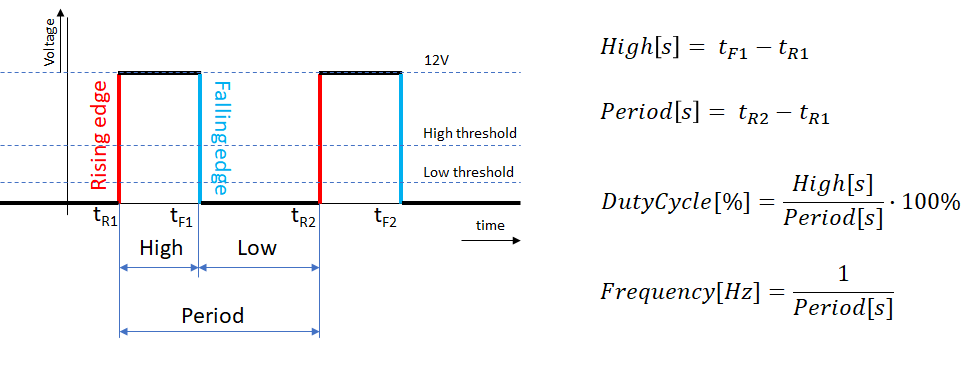The timer settings should follow requirements on frequency range and duty-cycle resolution. It is recommended that the timer settings allow detecting frequencies slightly beyond the required frequency range.

Let's consider an example of:

LimitSpecified frequency limitsDetected frequencyDetected period
Minimal PWM frequency20 Hz19.5 Hz0.051282 s
Maximal PWM frequency1000 Hz1010 Hz0.00099 s

Based on these limits, Timer settings can be calculated, considering the MCU clock settings and prescalers: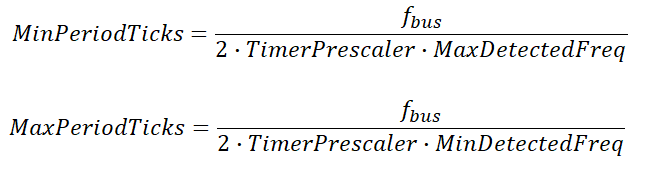For example, if the fbus = 50 MHz and the TimerPrescaler = 50, MinPeriodTicks for MaxDetectedFreq = 1010 Hz equals 495 ticks of the timer. The duty-cycle resolution at 1010 Hz is then 1/495, which is 0.202%. MaxPeriodTicks is key information for the timer settings since it needs to fit in the 16bit range with no overflow. In this case, the number of ticks is 25641 for 19.5Hz, which is suitable for 16-bit range with no issues. If the detected period (number of timer ticks) is outside of this range, the algorithm should go to the noSignalLevel state with defined noSignalOutput.

Finally, if the PWM signal is lost or corrupted, timer overflow event occurs and corresponding interrupt is called. Within the interrupt routine, the entire algorithm should be reset to wait for another rising edge, while turned in the noSignalLevel state with defined noSignalOutput.

## Selecting Detected State

Based on the PWM signal condition, frequency and duty-cycle detected, the algorithm state is selected. Following states are defined to help users with state identification:

StateDescriptionOutput
PWM_NoSignalDetectPWM signal is not detected or the PWM period is outside of the rangenoSignalOutput, usually zero or full command (according to the application requirements)
PWM_LowSignalDetectPWM signal is detected, the duty-cycle is within the noSignalLevel and hystLowSignalOn or hystLowSignalOff (depends on the hysteresis)lowSignalOutput, usually zero/stand-by
PWM_LinearCtrlDetectPWM signal is detected, the duty-cycle is within the hystLowSignalOn and linearSignalLevelMax

output = (dutyCycle * linearSignalSlope + linearSignalOffset) << linearSignalNshift,

from linearSignalOutputMin to linearSignalOutputMax

PWM_HighSignalDetectPWM signal is detected, the duty-cycle is higher than linearSignalLevelMaxlinearSignalOutputMax

## Linear Output Calculation

Linear output calculation is an easy way of interpolating two endpoints by a linear equation. In order to calculate the coefficients (slope and offset) and to improve the fixed point range, shifting is implemented as well.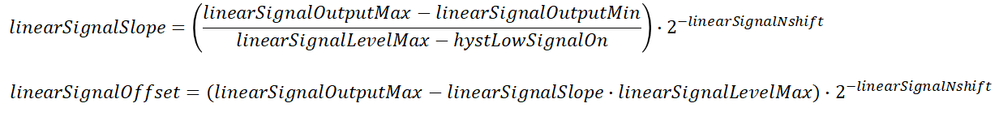# Algorithm Integration

## Interfaces

The algorithm is designed as a set of static functions with a structure of settings as a parameter to all the interfaces. This way, the same functionality can be used in multiple instances.

``````/* PWM Control data structure */
typedef struct
{
pwmControlStatus_t flags; //Control status bits
pwmControlConfig_t config; //Configuration
unsigned int period; //Modulation period
unsigned int dutyCycle; //dutyCycle
unsigned int hystDutyCycle; //previous hysteresis of duty cycle

#ifndef PWM_CONTROL_SW
unsigned int overflowCntr; //timer overflow counter
#endif
tFrac16 outputValue; //Output value
unsigned int ControlInputClass; //Classification of the input signal
} pwmControl_t;‍‍‍‍‍‍‍‍‍‍‍‍‍‍‍‍‍‍‍‍‍‍‍‍‍‍‍‍‍‍‍‍‍‍‍‍‍‍‍‍‍‍‍‍‍‍‍‍‍‍‍‍‍‍‍‍‍‍‍‍‍‍‍‍‍‍‍‍‍‍‍‍‍‍‍‍‍‍‍‍‍‍‍‍‍‍‍‍‍‍‍‍‍‍‍‍‍‍‍‍‍‍‍‍‍‍‍‍‍‍‍‍‍‍‍‍‍‍‍‍‍‍‍‍‍‍‍‍‍‍‍‍‍‍‍‍‍‍‍‍‍‍‍‍‍‍‍‍‍‍‍‍‍‍‍‍‍‍‍‍‍‍‍‍‍‍‍‍‍‍‍‍‍‍‍‍‍‍‍‍‍‍‍‍‍‍‍‍‍‍‍‍‍‍‍‍‍‍‍‍‍‍‍‍‍‍‍‍‍‍‍‍‍‍‍‍‍‍‍‍‍‍‍‍‍‍‍‍‍‍‍‍‍‍‍‍‍‍‍‍‍‍‍‍‍‍‍‍‍‍‍‍‍‍‍‍‍‍‍‍‍‍‍‍‍‍‍‍‍‍‍‍‍‍‍‍‍‍‍‍‍‍‍‍‍‍‍‍‍‍‍‍‍‍‍‍‍‍‍‍‍‍‍‍‍‍‍‍‍‍‍‍‍‍‍‍‍‍‍‍‍‍‍‍‍‍‍‍‍‍‍‍‍‍‍‍‍‍‍‍‍‍‍‍‍‍‍‍‍‍‍‍‍‍‍‍‍‍‍‍‍‍‍‍‍‍‍‍‍‍‍‍‍‍‍‍‍‍‍‍‍‍‍‍‍‍‍‍‍‍‍‍‍‍‍‍‍‍‍‍‍‍‍‍‍‍‍‍‍‍‍‍‍‍‍‍‍‍‍‍‍‍‍‍‍‍‍‍‍‍‍‍‍‍‍``````

The input-output characteristics are defined by a set of parameters as discussed earlier using the following structure:

``````typedef struct
{
/* No signal definition */
tFrac16 noSignalLevel; //Level of the duty cycle for NoSignal detection
tFrac16 noSignalOutput; //Output when NoSignal is detected
/* Low signal definition */
tFrac16 lowSignalOutput; //Output when LowSignal is detected
/* Linear signal definition */
tFrac16 hystLowSignalOff; //LowSignal-to-NoSignal hysteresis level
tFrac16 hystLowSignalOn; //NoSignal-to-LowSignal hysteresis level
tFrac16 linearSignalLevelMax; //Level of the duty cycle for LinearSignal saturation
tFrac16 linearSignalOutputMin; //Output at hystLowSignalOn
tFrac16 linearSignalOutputMax; //Output at linearSignalLevelMax
tFrac16 linearSignalSlope; // ((linearSignalOutputMax - linearSignalOutputMin)/(hystLowSignalOn - linearSignalLevelMax)))*2^(-linearSignalNshift)
tFrac16 linearSignalOffset; // (linearSignalOutputMax - (linearSignalSlope * linearSignalLevelMax))*2^(-linearSignalNshift)
tU16 linearSignalNshift; // scaling shift of the linear curve

/* timing settings */
unsigned int minPeriod; //minimal period of the signal to be detected, shorter period leads to PWM_NoSignalDetect
unsigned int maxPeriod; //maximal period of the signal to be detected, longer period leads to PWM_NoSignalDetect
} pwmControlConfig_t;‍‍‍‍‍‍‍‍‍‍‍‍‍‍‍‍‍‍‍‍‍‍‍‍‍‍‍‍‍‍‍‍‍‍‍‍‍‍‍‍‍‍‍‍‍‍‍‍‍‍‍‍‍‍‍‍‍‍‍‍‍‍‍‍‍‍‍‍‍‍‍‍‍‍‍‍‍‍‍‍‍‍‍‍‍‍‍‍‍‍‍‍‍‍‍‍‍‍‍‍‍‍‍‍‍‍‍‍‍‍‍‍‍‍‍‍‍‍‍‍‍‍‍‍‍‍‍‍‍‍‍‍‍‍‍‍‍‍‍‍‍‍‍‍‍‍‍‍‍‍‍‍‍‍‍‍‍‍‍‍‍‍‍‍‍‍‍‍‍‍‍‍‍‍‍‍‍‍‍‍‍‍‍‍‍‍‍‍‍‍‍‍‍‍‍‍‍‍‍‍‍‍‍‍‍‍‍‍‍‍‍‍‍‍‍‍‍‍‍‍‍‍‍‍‍‍‍‍‍‍‍‍‍‍‍‍‍‍‍‍‍‍‍‍‍‍‍‍‍‍‍‍‍‍‍‍‍‍‍‍‍‍‍‍‍‍‍‍‍‍‍‍‍‍‍‍‍‍‍‍‍‍‍‍‍‍‍‍‍‍‍‍‍‍‍‍‍‍‍‍‍‍‍‍‍‍‍‍‍‍‍‍‍‍‍‍‍‍‍‍‍‍‍‍‍‍‍‍‍‍‍‍‍‍‍‍‍‍‍‍‍‍‍‍‍‍‍‍‍‍‍‍‍‍‍‍‍‍‍‍‍‍‍‍‍‍‍‍‍‍‍‍‍‍‍‍‍‍‍‍‍‍‍‍‍‍‍‍‍‍‍‍‍‍‍‍‍‍‍‍‍‍‍‍‍‍‍‍‍‍‍‍‍‍‍‍‍‍‍‍‍‍‍‍‍‍‍‍‍‍‍‍‍‍‍‍‍‍‍‍‍‍‍‍‍‍‍‍‍‍‍‍‍‍‍‍‍‍‍‍‍‍‍‍‍‍‍‍‍‍‍‍‍‍‍‍‍‍‍‍‍‍‍‍‍‍‍‍‍‍‍‍‍‍‍‍‍‍‍‍‍‍‍‍‍‍‍‍‍‍‍‍‍‍‍‍‍‍‍‍‍‍‍‍‍‍‍‍‍‍‍‍‍‍‍‍‍‍‍‍‍‍‍‍‍‍‍‍‍‍‍‍‍‍‍‍‍‍‍‍‍‍‍‍‍‍‍‍‍‍‍‍‍‍‍‍‍‍‍‍‍‍‍‍‍‍‍‍‍‍‍‍‍‍‍‍‍‍‍‍‍‍‍‍‍‍‍‍‍``````

More details on the structures and values are described in the header file in attachment.

There is a set of exported functions to be integrated into the application.

``````/* Exported function headers */
extern void PWMControlInit(pwmControl_t * data); //Clears all the internal accumulators and prepars the algorithm for usage
extern unsigned int PWMControlUpdate(unsigned int pin, pwmControl_t * data); //To be called within the timer input capture interrupt, updates the internal values and calculates the PWM frequency and duty-cycle
extern tFrac16 PWMControlGetOutputValue(pwmControl_t * data); //To be called where necessary by the application to get the output value
extern void PWMControlTimerOverflow(pwmControl_t * data);‍‍‍‍‍ //To be called within the timer overflow interrupt to indicate that the signal is lost‍‍‍‍‍‍‍‍‍‍‍‍‍‍‍‍‍‍‍‍‍‍‍‍‍‍‍‍‍‍‍‍‍‍‍‍‍‍‍‍‍‍‍‍‍‍‍‍‍‍‍‍‍‍‍‍‍‍‍‍‍‍‍‍‍‍‍‍‍‍‍‍‍‍‍‍‍‍‍‍‍‍‍‍‍‍‍‍‍‍‍‍‍‍‍‍‍‍‍‍‍‍‍‍‍‍‍‍‍‍‍‍‍‍‍‍‍‍‍‍‍‍‍‍‍‍‍‍‍‍‍‍‍‍‍‍‍‍‍‍‍‍‍‍‍``````

## Integration

The PWM control source and header files should be included in the project to be compiled. The project uses NXP Automotive Math and Motor Control Library Set (AMMCLib version v1.1.17 or higher), which needs to be added to the project as well. For more information, please visit https://www.nxp.com/support/developer-resources/run-time-software/automotive-software-and-tools/auto...

The next step is to include the header file in the main application source code:

``#include "pwm_control.h"‍‍‍‍‍‍‍‍‍‍‍‍‍‍‍‍‍‍‍‍‍‍‍‍‍‍‍‍‍``

In order to provide necessary settings and to handle the output, the following global variables are recommended:

``````// PWM Control data
pwmControl_t pwmControlData; //PWM input control data structure
static tBool pwmControlEnabled = false; // Off by default‍‍‍‍‍‍‍‍‍‍‍‍‍‍‍‍‍‍‍‍‍‍‍‍``````

Device initialization should include clock settings, timer, and input pin routing settings accordingly. For S12ZVML device, the configuration of TIM, PIM and LIN modules is following:

Within the initialization part of the application, the following code should be placed to initialize the PWM control. Please consider the values as an example only.

``````// PWM control initial settings
pwmControlData.config.minPeriod = 495; //Period of the max frequency
pwmControlData.config.maxPeriod = 25641; //Period of the min frequency
pwmControlData.config.noSignalLevel = FRAC16(0.05); //No signal detected below this duty cycle
pwmControlData.config.noSignalOutput = FRAC16(0); //Output on ""no signal"" detected
pwmControlData.config.lowSignalOutput = FRAC16(0); //Output on ""low signal"" detected
pwmControlData.config.hystLowSignalOff = FRAC16(0.1); //Low level of the hysteresis. Smaller duty cycle means ""low signal"", higher duty cycle means ""switch on""
pwmControlData.config.hystLowSignalOn = FRAC16(0.15); //High level of the hysteresis. Smaller duty cycle means ""min speed or off"", higher duty cycle means ""switch on and linear""
pwmControlData.config.linearSignalLevelMax = FRAC16(0.85); //High level of the linear mode
pwmControlData.config.linearSignalOutputMin = FRAC16(0.116666666666667); //Output within the hysteresis mode
pwmControlData.config.linearSignalOutputMax = FRAC16(0.833333333333333); //Output at the linearSignalLevelMax
pwmControlData.config.linearSignalSlope = FRAC16(0.511904761904762); //Linear signal slope = (linearSignalOutputMax - linearSignalOutputMin) / (linearSignalLevelMax - hystLowSignalOn) * 2^(-linearSignalNshift)
pwmControlData.config.linearSignalOffset = FRAC16(-0.018452380952381); //Linear signal offset = (linearSignalOutputMin - (linearSignalOutputMax - linearSignalOutputMin) / (linearSignalLevelMax - hystLowSignalOn) * hystLowSignalOn) * 2^(-linearSignalNshift)
pwmControlData.config.linearSignalNshift = 1; //Linear signal shift
pwmControlData.config.f16pwmInputMA.u16NSamples = 5; //Moving average filter settings 2^n samples

PWMControlInit(&pwmControlData);‍‍‍‍‍‍‍‍‍‍‍‍‍‍‍‍‍‍‍‍‍‍‍‍‍‍‍‍‍‍‍‍‍‍‍‍‍‍‍‍‍‍‍‍‍‍‍‍‍‍‍‍‍‍‍‍‍‍‍‍‍‍‍‍‍‍‍‍‍‍‍‍‍‍‍‍‍‍‍‍‍‍‍‍‍‍‍‍‍‍‍‍‍‍‍‍‍‍‍‍‍‍‍‍‍‍‍‍‍‍‍‍‍‍‍‍‍‍‍‍‍‍‍‍‍‍‍‍‍‍‍‍‍‍‍‍``````

The configuration can be generated using an Excel sheet in the attachment. Content of the cell "D34" can be copied-pasted in the code. Name of the structure can be changed in "H1" cell. Orange-colored cells are customizable, while grey-colored cells use formulas to calculate output.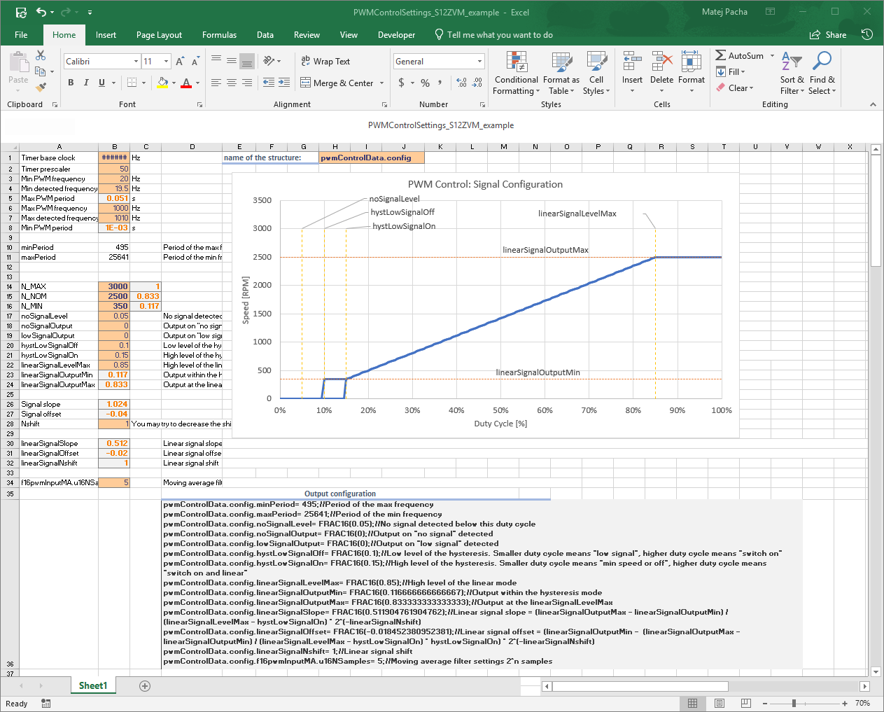Timer input capture interrupt routine should call the PWM control "Update" routine and switch the input capture mode between the rising edge and falling edge detection. In the case of S12ZVML device, the timer interrupt example follows:

``````INTERRUPT void TIM0chan3_ISR(void)
{
// Read PWM input control pin and update status
PWMControlUpdate(TIM0TC3, &pwmControlData);
// Read the PWM Control output
pwmControlData.outputValue = PWMControlGetOutputValue(&pwmControlData);

// Toggle edge detection
if(pwmControlData.flags.risingEdge)
{
TIM0TCTL4_EDG3A = 1;
TIM0TCTL4_EDG3B = 0;
}
if(pwmControlData.flags.fallingEdge)
{
TIM0TCTL4_EDG3A = 0;
TIM0TCTL4_EDG3B = 1;
}

// Clear interrupt flag
}‍‍‍‍‍‍‍‍‍‍‍‍‍‍‍‍‍‍‍‍‍‍‍‍‍‍‍‍‍‍‍‍‍‍‍‍‍‍‍‍‍‍‍‍‍‍‍‍‍‍‍‍‍‍‍‍‍‍‍‍‍‍‍‍‍‍‍‍‍‍‍‍‍‍‍‍‍‍‍‍‍‍‍‍‍‍‍‍‍‍‍‍‍‍‍‍‍‍‍‍‍‍‍‍‍‍‍‍‍‍‍‍‍‍‍‍‍‍‍‍‍‍‍‍‍‍‍‍‍‍‍‍‍‍‍‍‍‍‍‍‍‍‍‍‍‍‍‍‍‍‍‍‍‍‍‍‍‍‍‍‍‍‍‍‍‍‍‍‍‍‍‍‍‍‍‍‍‍‍‍‍‍‍‍‍‍‍‍‍‍‍‍‍‍‍‍‍‍‍‍‍‍‍‍‍‍‍‍‍‍‍‍‍‍‍‍‍‍‍‍‍‍‍‍‍‍‍‍‍‍‍‍‍‍‍‍‍‍‍‍‍‍‍‍‍‍‍‍‍‍‍‍‍‍‍‍‍‍‍‍‍‍‍‍‍‍‍‍‍‍‍‍‍‍‍‍‍‍‍‍‍‍‍‍‍‍‍‍‍‍‍‍‍‍‍‍‍‍‍‍‍‍‍‍‍‍‍‍‍‍‍‍‍‍‍‍‍‍‍‍‍‍‍‍‍‍‍‍‍‍‍‍‍‍‍‍‍‍‍‍‍‍‍‍‍‍‍‍‍‍‍‍‍‍‍‍‍‍‍‍‍‍‍‍‍‍‍‍‍‍‍‍‍‍‍‍‍‍‍‍‍‍‍‍‍‍‍‍‍‍‍‍‍‍‍‍‍‍‍‍‍‍‍‍‍‍‍‍‍‍‍‍‍‍‍‍‍‍‍‍‍‍‍‍‍‍‍‍‍‍‍‍‍‍‍‍‍‍‍‍‍‍‍‍‍‍‍‍‍‍‍‍‍‍‍‍‍‍‍‍‍‍‍‍‍‍‍‍‍‍‍‍‍‍‍‍‍‍‍‍‍‍‍‍‍‍‍‍‍‍‍‍‍‍‍‍‍‍‍‍‍‍‍‍‍‍‍‍‍‍‍‍‍‍‍‍‍‍‍‍‍‍‍‍‍‍‍‍‍‍‍‍‍‍‍‍‍‍‍‍‍‍‍‍‍‍‍‍‍‍‍‍‍‍‍‍‍‍‍‍‍‍‍‍‍‍‍‍‍‍‍‍‍‍‍‍‍‍‍‍‍‍‍‍‍‍‍‍‍‍‍‍‍‍‍‍‍‍‍‍‍‍‍‍‍‍‍‍‍‍‍‍‍‍‍‍‍‍‍‍‍‍‍‍‍‍‍‍‍‍‍‍‍‍‍‍‍‍``````

Timer overflow interrupt routine shall implement PWM control "Overflow" handler as follows (again, for S12ZVML device). Output value updater should be called as well to update the output value.

``````INTERRUPT void TIM0overflow_ISR(void)
{
// Detect PWM input control period overflow (ultra low frequencies
PWMControlTimerOverflow(&pwmControlData);
pwmControlData.outputValue = PWMControlGetOutputValue(&pwmControlData);
// Clear interrupt flag
}‍‍‍‍‍‍‍‍‍‍‍‍‍‍‍‍‍‍‍‍‍‍‍‍‍‍‍‍‍‍‍‍‍‍‍‍‍‍‍‍‍‍‍‍‍‍‍‍‍‍‍‍‍‍‍‍‍‍‍‍‍‍‍‍‍‍‍‍‍‍‍‍‍‍‍‍‍‍‍‍‍‍‍‍‍‍‍‍‍‍‍‍‍‍‍‍‍‍‍‍‍‍‍‍‍‍‍‍‍‍‍‍‍‍‍‍‍‍‍‍‍‍‍‍‍‍‍‍‍‍‍‍‍‍‍‍‍‍‍‍‍‍‍‍‍‍‍‍‍‍‍‍‍‍‍‍‍‍‍‍‍‍‍‍‍‍‍‍‍‍‍‍‍‍‍‍‍‍‍‍‍‍‍‍‍‍‍‍‍‍‍‍‍‍‍‍‍‍‍‍‍‍‍‍‍‍‍‍‍‍‍‍‍‍‍‍‍‍‍‍‍‍‍‍‍‍‍‍‍‍‍‍``````

Finally, within the application task, the PWM control data can be accessed and used in the application. For example, based on the ControlInputClass, the input signal processing may be enabled or disabled and the output value can be used as the required speed value of an engine cooling fan.

``````[Application Task]
{
// ...

// PWM Control
// If no signal is detected, enable manual switch (using FreeMASTER)
if(pwmControlData.ControlInputClass == PWM_NoSignalDetect)
{
if(pwmControlEnabled == true)
{
cntrState.usrControl.switchAppOnOff = 0;
pwmControlEnabled = false;
}
}
else
{
pwmControlEnabled = true;
}

// If PWM input control enabled, update the demanded speed
if(pwmControlEnabled)
{
//If the linear or high signal is detected, switch the app on
if(pwmControlData.ControlInputClass >= PWM_LinearCtrlDetect)
{
cntrState.usrControl.switchAppOnOff = 1;
//Store the demanded speed
drvFOC.pospeControl.wRotElReq = pwmControlData.outputValue;
}
//else switch off the app...
else
{
cntrState.usrControl.switchAppOnOff = 0;
//...and if a fault is indicated, clear the fault
if(cntrState.state == fault)
{
cntrState.usrControl.switchFaultClear = true;
}
}
}
// ...
}‍‍‍‍‍‍‍‍‍‍‍‍‍‍‍‍‍‍‍‍‍‍‍‍‍‍‍‍‍‍‍‍‍‍‍‍‍‍‍‍‍‍‍‍‍‍‍‍‍‍‍‍‍‍‍‍‍‍‍‍‍‍‍‍‍‍‍‍‍‍‍‍‍‍‍‍‍‍‍‍‍‍‍‍‍‍‍‍‍‍‍‍‍‍‍‍‍‍‍‍‍‍‍‍‍‍‍‍‍‍‍‍‍‍‍‍‍‍‍‍‍‍‍‍‍‍‍‍‍‍‍‍‍‍‍‍‍‍‍‍‍‍‍‍‍‍‍‍‍‍‍‍‍‍‍‍‍‍‍‍‍‍‍‍‍‍‍‍‍‍‍‍‍‍‍‍‍‍‍‍‍‍‍‍‍‍‍‍‍‍‍‍‍‍‍‍‍‍‍‍‍‍‍‍‍‍‍‍‍‍‍‍‍‍‍‍‍‍‍‍‍‍‍‍‍‍‍‍‍‍‍‍‍‍‍‍‍‍‍‍‍‍‍‍‍‍‍‍‍‍‍‍‍‍‍‍‍‍‍‍‍‍‍‍‍‍‍‍‍‍‍‍‍‍‍‍‍‍‍‍‍‍‍‍‍‍‍‍‍‍‍‍‍‍‍‍‍‍‍‍‍‍‍‍‍‍‍‍‍‍‍‍‍‍‍‍‍‍‍‍‍‍‍‍‍‍‍‍‍‍‍‍‍‍‍‍‍‍‍‍‍‍‍‍‍‍‍‍‍‍‍‍‍‍‍‍‍‍‍‍‍‍‍‍‍‍‍‍‍‍‍‍‍‍‍‍‍‍‍‍‍‍‍‍‍‍‍‍‍‍‍‍‍‍‍‍‍‍‍‍‍‍‍‍‍‍‍‍‍‍‍‍‍‍‍‍‍‍‍‍‍‍‍‍‍‍‍‍‍‍‍‍‍‍‍‍‍‍‍‍‍‍‍‍‍‍‍‍‍‍‍‍‍‍‍‍‍‍‍‍‍‍‍‍‍‍‍‍‍‍‍‍‍‍‍‍‍‍‍‍‍‍‍‍‍‍‍‍‍‍‍‍‍‍‍‍‍‍‍‍‍‍‍‍‍‍‍‍‍‍‍‍‍‍‍‍‍‍‍‍‍‍‍‍‍‍‍‍‍‍‍‍‍‍‍‍‍‍‍‍‍‍‍‍‍‍‍‍‍‍‍‍‍‍‍‍‍‍‍‍‍‍‍‍‍‍‍‍‍‍‍‍‍‍‍‍‍‍‍‍‍‍‍‍‍‍‍‍‍‍‍‍‍‍‍‍‍‍‍‍‍‍‍‍‍‍‍‍‍‍‍‍‍‍‍‍‍‍‍‍‍‍‍‍‍‍‍‍‍‍‍‍‍‍‍‍‍‍‍‍‍‍‍‍‍‍‍‍‍‍‍‍‍‍‍‍‍‍‍‍‍‍‍‍‍‍‍‍‍‍‍‍‍‍‍‍‍‍‍‍‍‍‍‍‍‍‍‍‍‍‍‍‍‍‍‍‍‍‍‍‍‍‍‍‍‍‍‍‍‍‍‍‍‍‍‍‍‍‍‍‍‍‍‍‍‍‍‍‍‍‍‍‍‍‍‍‍‍‍‍‍‍‍‍‍‍‍‍‍‍‍‍‍‍‍‍‍‍‍‍‍‍‍‍‍‍‍‍‍‍‍‍‍‍‍‍‍‍‍‍‍‍‍‍‍‍‍‍‍‍‍‍‍‍‍‍‍‍‍‍‍‍‍‍‍‍‍‍‍‍‍‍‍‍‍‍‍‍‍‍‍‍‍‍‍‍‍‍‍‍‍‍‍‍‍‍‍‍‍‍‍‍‍‍‍‍‍‍‍‍‍‍‍‍‍‍‍‍‍‍‍‍‍‍‍‍‍‍‍‍‍‍‍‍‍‍‍‍‍‍‍‍‍‍‍‍‍‍‍‍‍‍‍‍‍‍‍‍‍‍‍‍‍‍‍‍‍‍‍‍‍‍‍‍‍‍‍‍‍‍‍‍‍‍‍‍‍‍‍‍‍‍‍‍‍‍‍‍‍‍‍‍‍‍‍‍‍‍‍‍‍‍‍‍‍‍‍‍‍‍‍‍‍‍‍‍‍‍‍‍‍‍‍‍‍‍‍‍‍‍‍‍‍‍‍‍‍‍‍‍‍‍‍‍‍‍‍‍‍‍‍‍‍‍‍‍‍‍‍‍‍‍‍‍‍‍‍‍‍‍‍‍‍‍‍‍‍‍‍‍‍‍‍‍‍‍‍‍‍‍‍‍‍‍‍‍‍‍‍‍‍‍‍‍‍‍‍‍‍‍‍‍‍‍‍‍‍‍‍‍‍‍‍‍‍‍‍‍‍‍‍‍‍‍‍‍‍‍‍‍‍‍‍‍‍‍‍‍‍‍‍‍‍‍‍‍‍‍‍‍‍‍‍‍‍‍‍‍‍‍‍‍‍‍‍‍‍‍‍‍‍‍‍‍‍‍‍‍‍‍‍‍‍‍‍‍‍‍‍‍‍‍‍‍‍‍‍‍‍‍‍‍‍‍‍‍‍‍‍‍‍‍‍‍‍‍‍‍‍‍‍‍‍‍‍‍‍‍‍‍‍‍‍‍‍‍‍‍‍‍‍‍‍‍‍‍‍‍‍‍‍‍‍‍‍‍‍‍‍‍‍‍‍‍‍‍‍‍‍‍‍‍‍‍‍‍‍‍‍‍‍‍‍‍‍‍‍‍‍‍‍‍‍‍‍‍‍‍‍‍‍‍‍‍‍‍‍‍‍‍‍‍‍‍‍‍‍‍‍‍‍‍‍‍‍‍‍‍‍‍‍‍‍‍‍‍‍‍‍‍‍‍‍‍‍‍‍‍‍‍‍‍‍‍‍‍‍‍‍‍‍‍‍‍‍‍‍‍``````

# Example code

Example code is provided for S12ZVML-MINIKIT. The code is a modified devkit SW, which is available here (and related AN5327 here). The FreeMASTER project file is included with added PWM Control subblock. If there is no PWM signal detected, the application can be controlled via FreeMASTER on/off button. If the PWM signal is detected (even with a duty-cycle below the minimal value), the application listens and reacts as defined by the signal definition.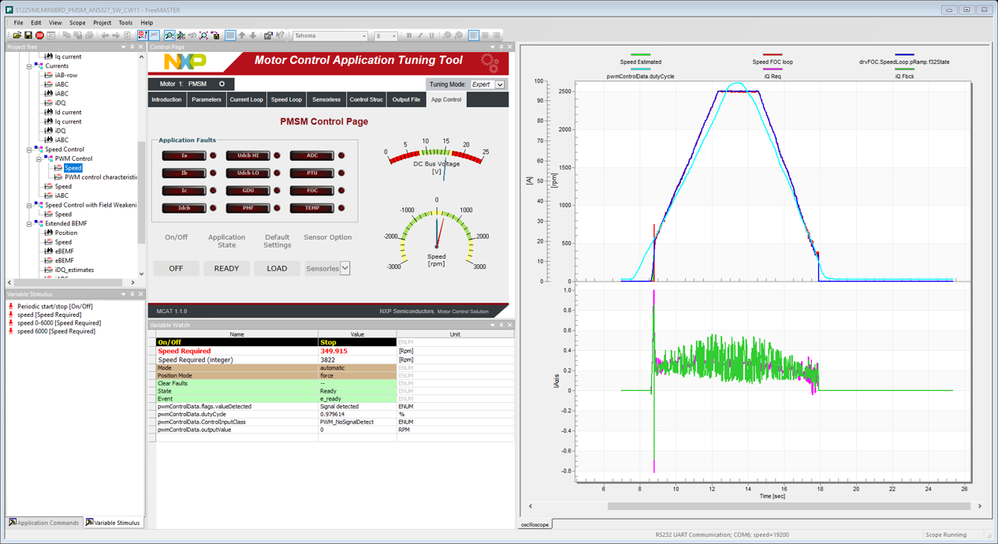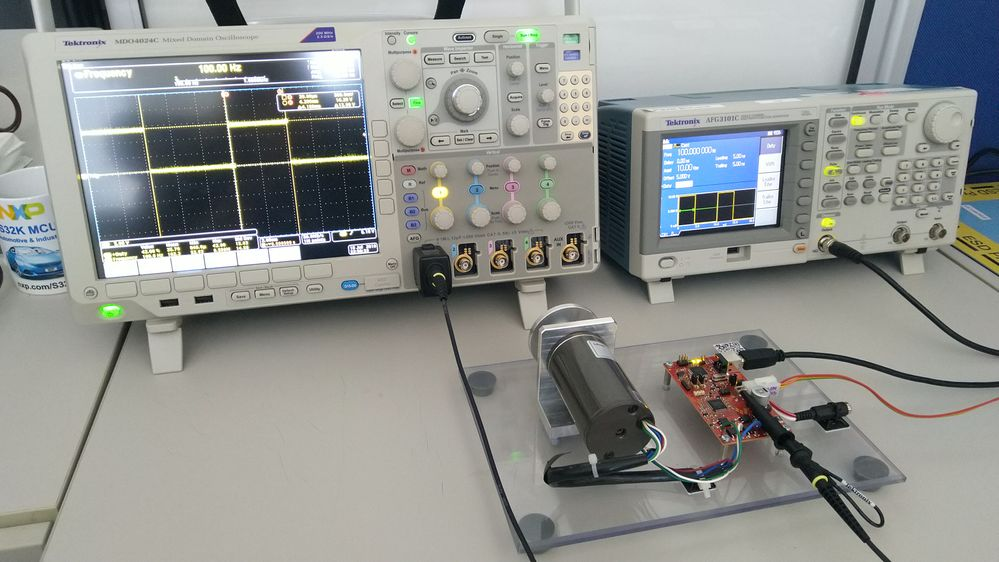# Conclusion

PWM control is still being used as one of the control signals to control auxiliary electric drives in automotive. Even if being replaced by LIN or CAN communication, there are still facilities which can benefit from software drivers enabling such a feature.

In this document, a software driver is provided to be integrated within the application. The example is provided for S12ZVML devices, however, it can be easily adopted to any device which meets the hardware requirements necessary for such PWM control signal detection.

Labels (1)
• ### General

Version history
Revision #:
2 of 2
Last update:
‎09-10-2020 02:10 AM
Updated by:Contributors